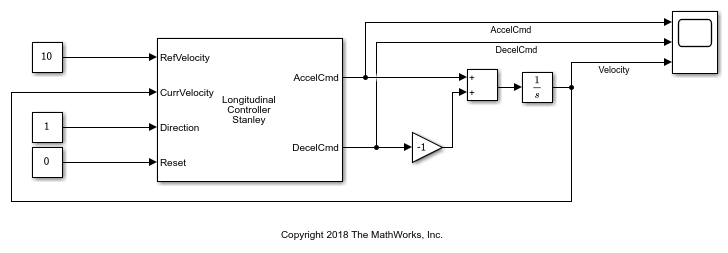Control Vehicle Velocity

This model uses a Longitudinal Controller Stanley block to control the velocity of a vehicle in forward motion. In this model, the vehicle accelerates from 0 to 10 meters per second.

The Longitudinal Controller Stanley block is a discrete proportional-integral controller with integral anti-windup. Given the current velocity and driving direction of a vehicle, the block outputs the acceleration and deceleration commands needed to match the specified reference velocity.Run the model. Then, open the scope to see the change in velocity and the corresponding acceleration and deceleration commands.The Longitudinal Controller Stanley block saturates the acceleration command at a maximum value of 3 meters per second. The Maximum longitudinal acceleration (m/s^2) parameter of the block determines this maximum value. Try tuning this parameter and resimulating the model. Observe the effects of the change on the scope. Other parameters that you can tune include the gain coefficients of the proportional and integral components of the block, using the Proportional gain, Kp and Integral gain, Ki parameters, respectively.